error: Content is protected !!

# PHYSICS: FORM THREE: Topic 7 - MEASUREMENT OF THERMAL ENERGY

TOPIC 7: MEASUREMENT OF THERMAL ENERGY

Heat capacity is the amount of heat required to raise the temperature of an object or substance by one degree. The temperature change is the difference between the final temperature ( Tf) and the initial temperature ( Ti).
The Factors which Determine Heat Quality of a Substance
Explain the factors which determine heat quality of a substance
Heat is a form of energy transferred between bodies due to difference in temperature between them. The energy possessed by the body due to its temperature is called the internal thermal energy. The heat content is due to the random motion of the particles that make up the body. The heat content of the substance depends on;
• mass of the substance
• temperature change
• specific heat capacity of the substance
The Heat Capacity
Determine the heat capacity
Heat capacity is the quantity of heat required to raise the temperature of an object by one degree Celsius.
Different objects have different heat capacities. That means, some can feel so hot than others for the same amount of heat energy supplied to them. For example, hot food on a metal plate feels very hot and difficult to touch than hot food on a plastic plate because a metal plate absorbs heat and raises its temperature faster than plastic plate. In this case we can say plastics have higher heat capacity than metals. In other words, it requires small amount of heat energy to raise temperature of metals than than in plastics.
If the object requires amount/quantity of heat energy Q to raise its temperature by one degree of centigrade, then the heat capacity C of the substance is obtained as;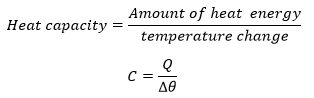Heat capacity can also be obtained mathematically by taking the product of mass of the substance and its specific heat capacity.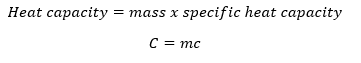Example 1
Find the heat capacity of a lump of copper of mass 50kg. The specific heat capacity of copper is 420 J/ Kg ºc.
Data Given
Mass of copper, m= 50kg
The specific heat capacity of copper, c = 420J/KgºC
Required: To calculate heat capacity, C.
C = mc
= 50Kg x 420J/KgºC
= 21600J
=21KJ
Calculating a quantity of heat
• The quantity of heat required to change the temperature of a body with mass, mkg byθ degree Celsius is mcθ joules.
• In order to raise the temperature of a body, heat must be supplied to it.
• In order to lower its temperature, heat must be removed from it.
The Heat Equation is therefore written:
Heat Gained or Heat Lost = Mass X specific heat capacity X change in temperature
Change in temperature:
H =mcθ
Where
H=Heat gained / lost
m= Mass of the body
θ= change (Rise or fall) In Temperature of the body.
Example 2
Water of mass 3kg is heated from 26ºc to 96ºC. Find the amount of heat supplied to the water given that the specific heat capacity of water is 4.2 x 103 J / Kg ºc
Data Given
Mass of water, m = 3kg
Specific Heat capacity, c = 4.2 X 103 j / kgº C
Initial temperature, θi = 26 ºC
Final Temperature, θf = 96ºC
Required
The amount of heat, H
H= mcθ
c = H/mθ
H = mcθ
H = 3Kg x 4.2 x103 (96-26) ºC
The amount of heat energy is H= 882000J= 882KJ

The Specific Heat Capacity
Determine the specific heat capacity
Specific heat capacity is the quantity of heat required to raise the temperature of a unit mass of a substance by one degree Celsius.
The quantity of heat supplied to or taken away from a body depends on:
1. The mass of the body, M
2. The temperature different, ΔT
3. The thermal properties of the body.
Transfer of Heat
Heat energy tends to flow from High temperatures to Low temperatures
If you pick up a warm object, heat energy transfers from the object to your hands and your hands feel warm. If you pick up a cool object, heat energy transfers from hands to the object and your hands feel cold.
Determining specific Heat capacity
• Calorimeter – Is the special instrument or vessel used for measurement of Heat.
• Calorimeter is highly polished metal can usually made of copper or aluminium.
• It is flitted with an insulating cover in which there are two holes.
• Two holes allow a thermometer and a stirrer to be inserted.
• The stirrer is made of the same metal as that of the calorimeter.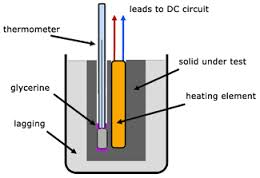Demonstration of the specific Heat capacity of a solid
Determining specific Heat capacity by Method of Calculation.
Heat lost by solid, Hs = Ms x Cs (Qs – Qf)
Heat Gained by Calorimeterand stirrer, Hc = Mc x Cc (Qf – Qi)
Heat Gained by Water, HW = Mw x Cw (Qf – Qi)
But the heat lost by the solid is equal to heat gained by the calorimeter and stirrer plus the heat gained by the water in the calorimeter.
Hs = Hc + Hw
But
Heat gained by a calorimeter and content equal to heat lost by the solid.
Thus Hc + Hl = Hs
Mc Cc (Qf – Qi) + Mi Ci ( Qf-Qi) = Ms Cs ( Qs-Qs)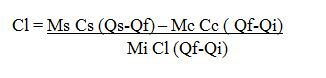Example 3
A piece of metal with a mass of 200g at a temperature of 100ºC is quickly transferred into 50g of water at 20ºC find the final temperature of the system ( specific Heat capacity of water Cw = 4200J/ Kg ºC specific Heat capacity of the metal Cm = 400J/KgºC.
Ms Cs (Qs-Qf) = Mc Cc (Qf-Q) Mm Cw (Qf-Ql)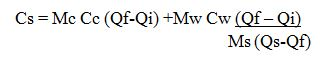Where:
Cs. Is the specific Heat capacity of the solids.
Determining the specific heat capacity of liquid, Cl
By calculation method;
Heat Gained by calorimeter and stirrer
Hc = Mc Cc (Qf – Qi)
Heat Gained by liquid
Hi = ML CL (Qf – Qi)
Heat lost by the solid
Hs = Ms Cs ( Qs-Qf)
Let
Q be the final Temperature of the system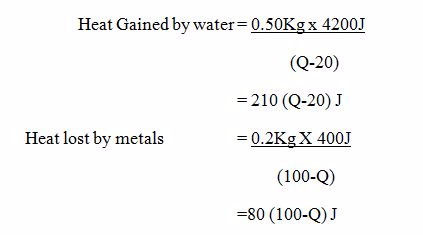If there are no heat Losses to the surroundings, then.
(Heat gained by water) = (Heat lost by metal)
210 (Q-20) = 80(100 – Q)
21(Q-20) = 8 (100-Q)
21Q – 420 = 800 – 8Q
21Q +8Q = (800+420)
29Q = 1220
Q = (1220/29)
Q = 42.1ºC
Change of state is the transformation of the condition of matter from one (state) to another caused by the change In temperature.
The Behaviour of Particles of Matter by Applying Kinetic Theory
Explain the behaviour of particles of matter by applying kinetic theory
The kinetic theory of matter (particle theory) says that all matter consists of many, very small particles which are constantly moving or in a continual state of motion. The degree to which the particles move is determined by the amount of energy they have and their relationship to other particles. The particles might be atoms, molecules or ions. Use of the general term 'particle' means the precise nature of the particles does not have to be specified.
Particle theory helps to explain properties and behaviour of materials by providing a model which enable us to visualise what is happening on a very small scale inside those materials. As a model, it is useful because it appears to explain many phenomena but as with all models it does have limitations.
Solids, liquids and gases
 In solids the particles In liquids the particles In gases the particles are held tightly and packed fairly close together - they are strongly attracted to each other o are in fixed positions but they do vibrate are fairly close together with some attraction between them are able to move around in all directions but movement is limited by attractions between particles have little attraction between them are free to move in all directions and collide with each other and with the walls of a container and are widely spaced out
The model can be used to help explain:
1. the properties of matter
2. what happens during physical changes such as melting, boiling and evaporating
The properties of matter
SolidsLiquidsGases
• have a definite shape
• maintain that shape
• are difficult to compress as the particles are already packed closely together
• are often dense as there are many particles packed closely together
• do not have a definite shape
• flow and fill the bottom of a container. They maintain the same volume unless the temperature changes
• are difficult to compress because there are quite a lot of particles in a small volume
• are often dense because there are quite a lot of particles in a small volume
• do not have a definite shape
• expand to fill any container
• are easily compressed because there are only a few particles in a large volume
• are often low density as there are not many particles in a large space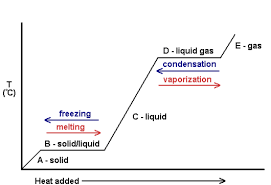The graph of temperature versus temperature for a Heated.

The Melting Point of a Substance from its Cooling Curve
Determine experimentally the melting point of a substance from its cooling curve
Melting is the process of change of the state of matter from solid into liquid e.g ice into water.
Melting point (M.P): It is the temperature at which solid substance tends to change into liquid.
Freezing: It is the process of change of the state of matter from liquid to solid e.g water into ice.
Freezing point: Is the temperature at which liquid change into solid. E.g water change into ice at OºC.
Evaporation:Is the process of change liquid substance into vapour (gas).
Sublimation: It is the change of state of matter from solid to gas and vice versa without passing through the liquid phase.e.g. Ammonium Chloride ( NH4CL) and Iodine tends to sublime.
Sublimation point is the temperature at which a solid tends to change into gas and vice versa without passing through liquid state.
Condensation:Is the change of state of gaseous state of matter into liquid state.e.g steam into water.
Deposition: Is the change of the state matter from gas into solid. e.g. Ammonium chloride vapour and Iodine vapour into solid (NH4CI) and (Iodine).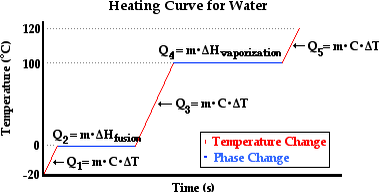Demonstration of cooling and melting curves for (octadecanoic acid).
Melting point (m.p) table
 Substance Melting point (ºC) Copper 1083 Glass 1000 – 1400 Iron 1450 Lead 327 Pitch 40 – 80 Mercury - 39 Platinum 1775 Tin 232 Tungsten 3377

The Effect of Impurities on the Freezing Point and the Boiling Point of a Substance
Demonstrate the effect of impurities on the freezing point and the boiling point of a substance
The effect of dissolved substances on the boiling point and melting point (M.P) means that the additional of impurities will result in increased (B.P) and (M.P).
Effect of impurities on Boiling Point
When an impurity is added to a substance its boiling point is elevated, i.e., its boiling point is increased.
The elevation in boiling point increases with increase in concentration of the solute because when adding the solute vapour pressure of the solution becomes lower than pure solvent. Thus the solution has to be heated more to make the vapour pressure equal to atmospheric pressure. Thus the boiling point gets elevated.
For example boiling point of water is 100oC under normal atmospheric pressure. If we add sugar or salt to this water its vapour pressure becomes lower and boiling point increases.
Generally, when 1 mole of any non electrolyte is dissolved in 1 litre of water the elevation of boiling point is 0.530.
Effect of impurities on freezing point
When an impurity is added its freezing point is lowered i.e. its freezing point decreases.
The depression in freezing point increases with the increase in concentration of the solute because on adding the solute the vapour pressure of solution becomes lower than that of pure solvent. Since freezing point is the temperature at which vapour pressure of liquid and solid phase are equal, therefore, for the solution, this will occur at a lower-temperature.
For example the freezing point of water is OoC under normal atmospheric pressure. If we add sugar or salt to this water its vapour pressure lowers and freezing point decreases.
Generally, when 1 mole of any non-electrolyte is dissolved in 1 litre of water the depression in freezing point of water is 1.860C.
Conclusion
1. The impurities present in a liquid pull its two fixed points away from each other i.e. the freezing point is lowered while the boiling point is raised.
2. The depression in freezing point and the elevation in boiling point increases with increase in the concentration of the solute or impurity i.e. these are the colligative properties that depends only on the no. of moles of the solute. They are independent of the nature of the solute.

The Effect of Pressure on the Boiling Point and Freezing Point of a Substance
Demonstrate the effect of pressure on the boiling point and freezing point of a substance
If a substance expands on solidifying, e.g., water, then the application of pressure lowers its melting point.
If a substance contracts on freezing, the pressure raises its melting point, e.g., paraffin wax.
The freezing point of water is lowered by 0 .007 ºC per atmosphere increase in pressure, whereas that of paraffin wax increases by 0.04 ºC per atmosphere increase in pressure.
When a is liquid heated, its temperature rises and eventually remains constant.
Boiling is the process of forming bubbles of vapour inside the body of a liquid. It rises to the surface of liquid. The process usually depends onexternal pressure above the liquid.

The Phenomenon of Regelation
Explain the phenomenon of regulation
Regelation is the Refreezing process which takes place when copper wire is passed through the Ice BLOCK
Regelation is the Refreezing process which takes place when the wire is observed to Cuts right through the ice block and falls on the floor.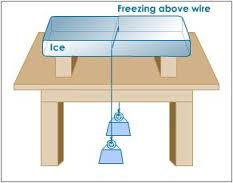The Concept of Boiling and Evaporation in Respect to the Kinetic Theory of Matter
Give the concept of boiling and evaporation in respect to the kinetic theory of matter
If a liquid is heated, the particles are given more energy and move faster and faster expanding the liquid. The most energetic particles at the surface escape from the surface of the liquid as a vapour as it gets warmer. Liquids evaporate faster as they heat up and more particles have enough energy to break away. The particles need energy to overcome the attractions between them. As the liquid gets warmer more particles have sufficient energy to escape from the liquid. Eventually, even particles in the middle of the liquid form bubbles of gas in the liquid. At this point the liquid is boiling and turning to gas. The particles in the gas are the same as they were in the liquid except that they have more energy. At normal atmospheric pressure, all materials have a specific temperature at which boiling occurs. This is called the "boiling point" or boiling temperature. As with the melting point, the boiling point of materials vary widely, e.g., nitrogen -210°C, alcohol 78°C, and aluminium 459°C.
Any material with a boiling temperature below 20°C is likely to be a gas at room temperature. When liquids boil the particles must have sufficient energy to break away from the liquid and to diffuse through the surrounding air particles. As these particles cool down and lose energy they will condense and turn back to liquid. When steam is formed by water boiling at 100°C the particles quickly condense as the surrounding air temperature is likely to be much less that 100°C so the particles cool rapidly. In fact the "steam" coming out of a boiling kettle can only be seen because some of the gas particles have condensed to form small droplets of water.
Evaporating
Within a liquid some particles have more energy than others. These "more energetic particles" may have sufficient energy to escape from the surface of the liquid as gas or vapour. This process is called evaporation and the result of evaporation is commonly observed when puddles or clothes dry. Evaporation takes place at room temperature which is often well below the boiling point of the liquid. Evaporation happens from the surface of the liquid. As the temperature increases, the rate of evaporation increases. Evaporation is also assisted by windy conditions which help to remove the vapour particles from the liquid so that more escape.
Evaporation is a complex idea for children for a number of reasons. The process involves the apparent disappearance of a liquid which makes the process difficult for them to understand. It is not easy to see the water particles in the air. Also, evaporation occurs in a number of quite differing situations - such as from a puddle or bowl of water where the amount of liquid obviously changes, to situations where the liquid is less obvious - such as clothes drying or even those where there is no obvious liquid at all to start with - such as bread drying out. A further complication is that evaporation may be of a solvent from a solution e.g. water evaporating from salt water to leave salt. These situations are quite different yet all involve evaporation.
Evaporation may also involve liquids other than water e.g. perfume, petrol, air fresheners. The particle model can be used to explain how it is possible to detect smells some distance away from the source.

Latent Heat of Fusion and Vaporisation
Demonstrate latent heat of fusion and vaporisation
Latent Heat is the energy when is supplied in form of heat required to change the state of the Matter from one form into another.
Latent heat is not determined (detected) by using a thermometer. So latent heat is also called hidden heat.
Specific latent Heat is the energy supplied to a unit Mass and change Its state from one state of Matter to another state of matter.
Latent heat of Vaporization is the heat required to change a liquid into a gaseous state at constant temperature.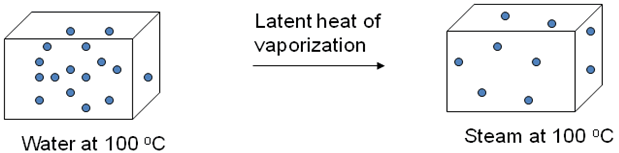• Mass of Beaker = Mkg
• Mass of Beaker + Water = M2 kg
• Time taken to Boil =tMinutes
• Time taken to Boil away = tMinutes
• Specific latent heat of = L J / kg Vapor
• Heat gained by steam = (M2 – M1)L
Generally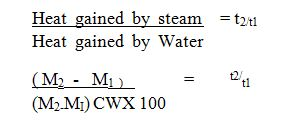Ttime taken to evaporate
T2 time taken to boil
In this experiment , the Heat gained by the Beaker may be Neglected.
Latent heat of fusion is the amount of heat required to change a substance from solid to liquid at constant temperature.
Example 4
Calculate the amount of Heat required to melts 800g of Ice at 0ºC The specific Latent of fusion of Ice 33400J/kg
Data given:
Mass of Ice , M = 800g (0.8kg)
Specific Heat of fusion, L = 33400 J/kg
Heat gained, H = ML
H= ( 0.8 x 33400J/ kg)
H = 267520J
Determination of the specific Latent Heat of fusion of Ice.
• Mass of Calorimeter + stirrer = M1
• Mass of calorimeter +Water =M2
• Mass of Calorimeter +Water = M3
• Initial Temperature of Water = Q1
• Final temperature of Water =Qf
• Mass of Water = ( M2 - M1 )
• Mass of Ice = ( M3 - M2 )
The Ice melts and forms Water at 0ºC .The Water formed warm up to Temperature Qf.Heat gained by ice during melting at 0ºC = (M3 - M2)L where L is the specific latent Heat of fussion.
Heat gained by the water formed = (M3 - M2) CW QF
Where
• CW is the specific heat capacity of water.
• Heat lost by the original water in the calorimeter = (M- M1) ( Q1 - QF ) Cw.
• heat lost by calorimeter and stirrer = M1 CC ( Qi - Qf ).
• Cc is the specific heat capacity of the material of the calorimeter.
Applying the heat equation:
(Heat gained by ice in Melting + Heat gained by the Water formed) =(Heat lost by calorimeter and stirrer + Heat lost by original Water)
( M3 - M2) L + (M3- M2) CW QF= M1 CC (Q1 - QF)+ (M2- M1 CW Q1 - QF)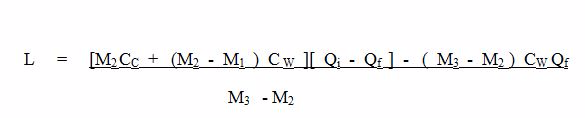Specific Latent heat of Vaporisation is the amount of heat required to change a unit Mass of liquid into gaseous state ( Vapour) at constant temperature.
Specific latent Heat of fusion is the amount of heat required to change a unit Mass of solid substance into liquid at constant temperature
 SUBSTANCE SPECIFIC LATENT HEATOF FUSSION J/ kg Ice 334400 Naphthalene 146300 Lead 24662 Copper 179740 Aluminum 317680 Gold 66880
Example 5
0.6 kg of ice at - 10ºC is dropped into 2kg of Water 49ºC contained in a Copper calorimeter of mass 0. 15kg . If the final temperature of the Mixture is 20ºC fin d the specific latent Heat of fusion of ice.
Where
• Specific Heat capacity of ice = 2.1 x 103 J/ KgºC
• Specific Heat capacity of copper = 420 J/ Kg ºC
• Specific Heat Capacity of Water = 4200 j/ Kg º C
Solution
Heat gained by ice during warming up form - 10 ºC to 0ºC
= ( 0 . 6 ×2 . 1 X 103 ×10)
= 12600J
Heat gained when ice at 0ºC changes to water at 0ºC = 0.6L; where L is the latent heat of fusion of ice
Heat gained by cold Water in warming up from 0ºC to 20ºC
=( 0 . 6 ×4 . 2 × 103 × 20)
=50400 J
Heat lost by Water during cooling from 49ºC to 20ºC
= 0 . 15 ×420 ×29
= 1827 J
But
Total Heat gained = Total Heat lost
12600 + 0.6 L + 50400 = 243600 + 1827
L = 245427 - 6300\ O.6
L = 304045J/ Kg

The Mechanism of Refrigeration
Describe the mechanism of refrigeration
Refrigerator is a machine which can enable Heat to flow from a cold Region to a Hot region.The Basic principle used in Refrigeration is Cooling by absorption of latent Heat
How it Works
A volatile liquid such as freon, evaporates inside the copper coils surrounding the freezing cabinet or the refrigeration.
• The latent heat of Vaporization comes from the air surrounding the coil i.e. from the inside of the freezing g cabinet
• An eclectically driven pump P remove the vapor from A and force it into the heat exchanger C, which is made of copper coils.
• The coils of the heat exchanger are filled with cool fins F
• In the heat exchanger, vapor is compressed by the pump and condensed back to liquid.
• The conversion of vapour into liquid in (c) gives out the latent heat of vaporization, which is conducted away by the fins.
• The condensed liquid is then returned to the evaporator coil (A) through avalve (V) (in this way a continuous circulation of vapour and liquid is set up).
• The rate of evaporation and the degree of cooling is controlled by a thermostat, which switches the pumps motor on and off at intervals.
• The thermostat can be adjusted to give the desired low temperature inside the freezing cabinet where food is preserved.